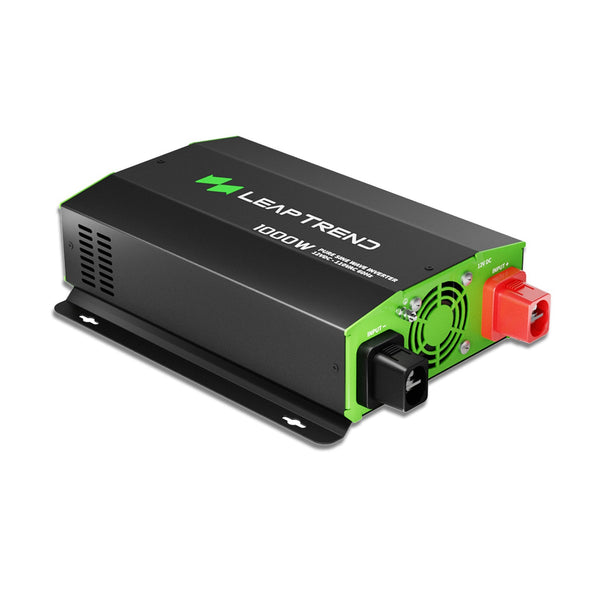# Inverter Unit Consumption

Introduction: An inverter is an electronic device used to convert direct current (DC) into alternating current (AC). Understanding the power consumption of inverters is crucial when selecting one. This article will explain the concept of inverter unit consumption and provide examples of power consumption for different power inverters.

## What is Inverter Unit Consumption?

Inverter unit consumption refers to the energy consumed by an inverter during operation. It is typically measured in watts (W) or kilowatt-hours (kWh). The magnitude of pure sine wave inverter unit consumption depends on the inverter's power and efficiency.## Factors Affecting Inverter Unit Consumption

The power consumption of an inverter unit depends on various factors, such as the size of the unit, climate, thermostat setting, and other factors. A typical 1500W inverter AC will use roughly 60 units of power (Pa) per hour, while a non-inverter 1000W AC will use about 47 Pa/hr. Inverter ACs consume at least 5-10% less power when compared with non-inverter ACs. A study comparing the energy consumption of a regular and an inverter AC of the same capacity in a typical office room under similar operating conditions found that the daily average energy consumption (for an 8-hour operating period) was 13.5 kWh for the standard AC and 8.7 kWh for the inverter type AC.Several factors influence inverter unit consumption, including but not limited to:

Design and manufacturing quality of the inverter

Operating temperature and environmental conditions